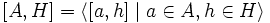Commutator of a normal subgroup and a subset implies 2-subnormal

This article describes a computation relating the result of the commutator operator on two known subgroup properties or properties of subsets of groups: (i.e., normal subgroup and subset of a group), to another known subgroup property (i.e., 2-subnormal subgroup)
View a complete list of commutator computations
This article gives the statement and possibly, proof, of an implication relation between two subgroup properties. That is, it states that every subgroup satisfying the first subgroup property (i.e., subgroup realizable as the commutator of a normal subgroup and a subset) must also satisfy the second subgroup property (i.e., 2-subnormal subgroup)
View all subgroup property implications | View all subgroup property non-implications
Get more facts about subgroup realizable as the commutator of a normal subgroup and a subset|Get more facts about 2-subnormal subgroup

Statement

Suppose$G$ is a group,$H$ is a normal subgroup of$G$, and$A$ is any subset of$G$. Then, the subgroup:$[A,H] = \langle [a,h] \mid a \in A, h \in H \rangle$

is a 2-subnormal subgroup of$G$.

Facts used

1. Subgroup normalizes its commutator with any subset: If$H \le G$ and$A$ is a subset of$G$,$H$ normalizes$[A,H]$.

Proof

Given: A group$G$, a normal subgroup$H$ of$G$, a subset$A$ of$G$.

To prove:$[A,H]$ is 2-subnormal in$G$.

Proof:

1. (Given data used:$H$ is normal in$G$): Since$H$ is normal in$G$,$[G,H] \le H$. Thus,$[A,H] \le H$.
2. (Fact used: fact (1)): By fact (1),$H$ normalizes$[A,H]$. Combining this with step (1), we get that$[A,H]$ is normal in$H$.
3. We thus have that$[A,H]$ is normal in$H$, and$H$ is normal in$G$. Thus,$[A,H]$ is 2-subnormal in$G$.